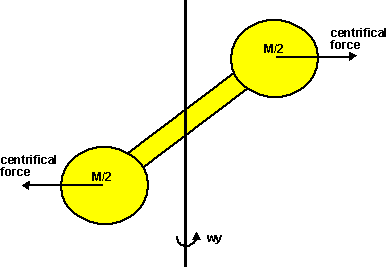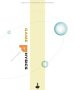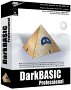# Physics - Newtons first law

This is an example to help me understand the Newton / Euler laws.

Imagine a rigid object rotating in free space, since there are no external forces acting on it then by the first law:

• Linear momentum in x dimension = constant.
• Linear momentum in y dimension = constant.
• Linear momentum in z dimension = constant.
• Angular momentum about x axis = constant.
• Angular momentum about y axis = constant.
• Angular momentum about z axis = constant.

Imagine the object is shaped as follows, for simplicity assume most of its mass is concentrated at its ends:Assume it is rotating about the y axis (wx = wz = 0). Then centifical forces would be generated which would tend to rotate it until the masses are aligned at 90 degrees to the y axis. If this happened then the rotation would slow down to keep wy * Iyy constant.

But what I don't understand is: while it is making this transition it must have some instantaneous rotation in the wx and wz directions? which appears to contradict the conservation laws? or does it ? in the position shown it would have an apparent clockwise rotation in the z axis, but then when it has rotated 180 degrees in the y axis it appears to be rotating anti-clockwise in the z axis. So perhaps this transition does not involve rotation about x and z?

Or is this a gyroscope issue? and will precession type forces be generated to cancel out the cenrifical forces and will it continue to rotate off axis?

So how can I write equations for the motion of an object without any external forces?

I originally thought that I could define the motion with the constants vx,vy,vz, wx,wy and wz. Where v is the velocity of the centre of mass and w is the rotation about the centre of mass. However this does not seem to be sufficient to describe this movement?

The conservation laws don't appear to be enough to describe what is happening here? its as if some other quantity is being minimised or maximised?

Can anyone help clarify my thinking?

This example is to help me understand the concepts of dynamics here

Where I can, I have put links to Amazon for books that are relevant to the subject, click on the appropriate country flag to get more details of the book or to buy it from them.Game Physics - This book has some useful stuff, its more of a textbook, not a step by step guide (although it does have a disc with a lot of C++ code). About the first third of the book is a physics textbook with theoretical exercises, the middle bit covers physics engine topics, and the last third of the book covers mathematical topics. I think I would use this book as a reference book to lookup the theory behind something I might be working on rather than a book to work through in order.

Commercial Software Shop

Where I can, I have put links to Amazon for commercial software, not directly related to the software project, but related to the subject being discussed, click on the appropriate country flag to get more details of the software or to buy it from them.Dark Basic Professional Edition - It is better to get this professional editionThis is a version of basic designed for building games, for example to rotate a cube you might do the following: make object cube 1,100 for x=1 to 360 rotate object 1,x,x,0 next x

This site may have errors. Don't use for critical systems.